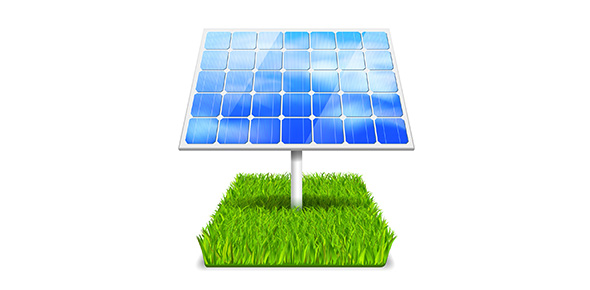# Quiz On Forms Of Energy And Resources

15 Questions | Attempts: 1375
ShareSettings• 1.
Skateboard rolling downhill is an example of what form of energy?
• A.

Chemical energy

• B.

Electromagnetic energy

• C.

Mechanical energy

• 2.
The light shining from a light bulb is an example of what form of energy?
• A.

Electromagnetic energy

• B.

Thermal energy

• C.

Chemical energy

• 3.
Lightning during a thunderstorm is an example of what form of energy?
• A.

Thermal energy

• B.

Electrical energy

• C.

Chemical energy

• 4.
Energy stored in food is an example of what form of energy?
• A.

Thermal energy

• B.

Chemical energy

• C.

Electrical energy

• 5.
Heat given off by a fire is an example of what form of energy?
• A.

Mechanical energy

• B.

Thermal energy

• C.

Nuclear energy

• 6.
Solar energy is energy from the sun.
• A.

True

• B.

False

• 7.
PV cells turn light from the sun into heat.
• A.

True

• B.

False

• 8.
Wind power works by having the wind turn a turbine to produce electricity.
• A.

True

• B.

False

• 9.
Hydropower (moving water) works by getting electrons out of the water.
• A.

True

• B.

False

• 10.
Geothermal energy can be used anywhere on Earth.
• A.

True

• B.

False

• 11.
Geothermal energy produces very little or no pollution.
• A.

True

• B.

False

• 12.
Trees (or wood) are the most common form of biomass in most areas.
• A.

True

• B.

False

• 13.
Oil is usually formed from the remains of living things that lived in the _____________.
• 14.
_____________ transport natural gas from its source to where it's used.
• 15.
Coal is a ____________ fossil fuel formed from plant remains.
• A.

Liquid

• B.

Solid

• C.

Gas

## Related TopicsBack to top
×

Wait!
Here's an interesting quiz for you.